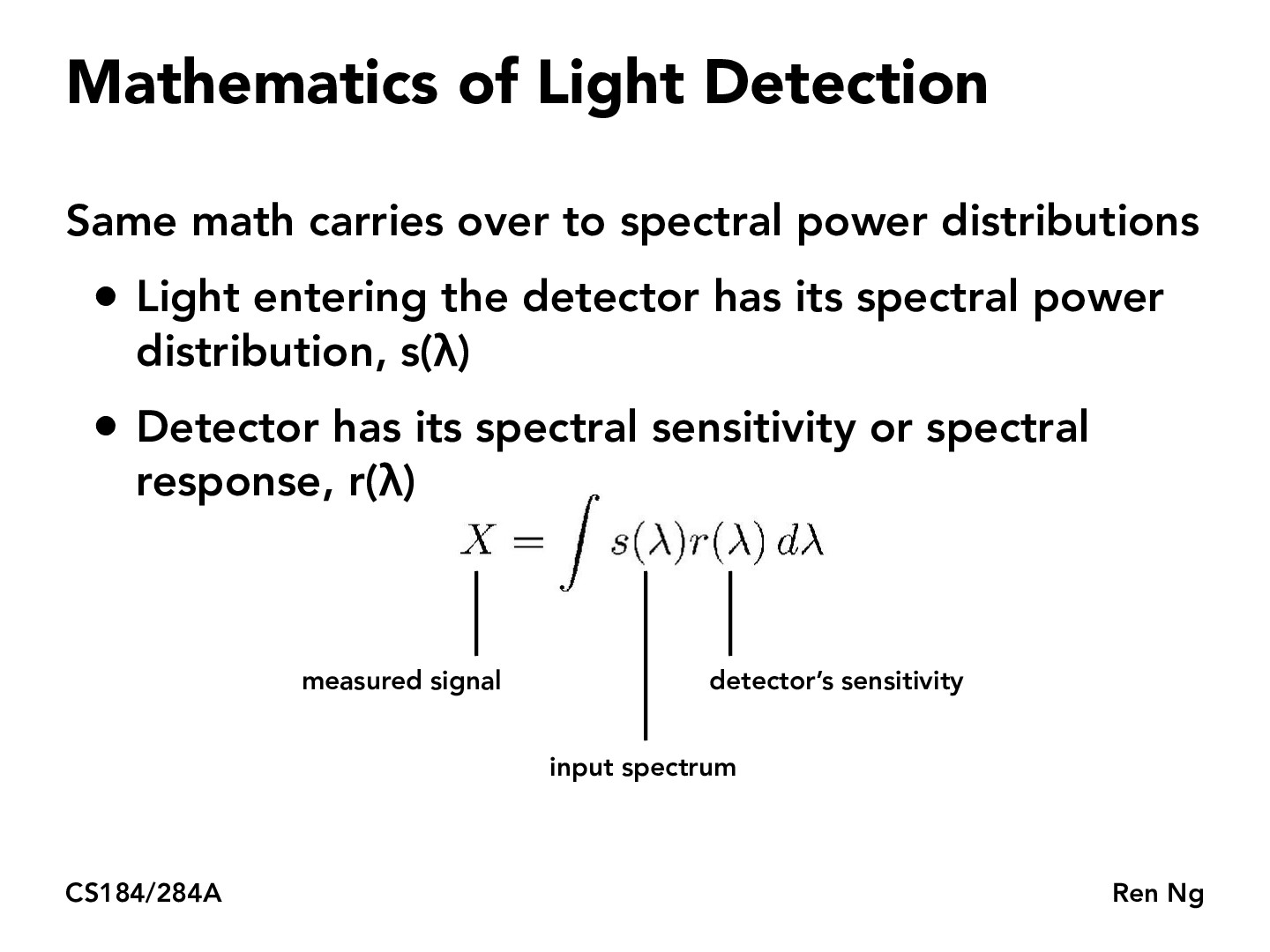You are viewing the course site for a past offering of this course. The current offering may be found here.Lecture 19: Introduction to Color Science (34)jgforsberg

Is the measured signal similar to the expected value of the detector? The formula looks very similar to the expected value for a continuous distribution we saw earlier in the semester.zehric

it might also be similar to convolutionmoridin22

There isn't really an expected value here since we don't have any randomness, but if we introduced some randomness into the system (like only sending one wavelength at a time with pdf given by the s function) then it could be similar!yzyz

We can kind of think of this as an expected value, if we think of the detector sensitivity $r(\lambda)$ as the "probability" that the detector detects light with wavelength $\lambda$.

You must be enrolled in the course to comment